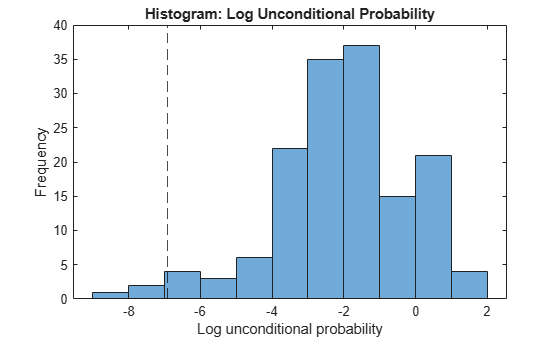# logP

Log unconditional probability density for naive Bayes classifier

## Syntax

``lp = logP(Mdl,tbl)``
``lp = logP(Mdl,X)``

## Description

````lp = logP(Mdl,tbl)` returns the log unconditional probability density of the observations (rows) in `tbl` using the naive Bayes model `Mdl`.You can use `lp` to identify outliers in the training data.```

example

````lp = logP(Mdl,X)` returns the log unconditional probability density of the observations (rows) in `X` using the naive Bayes model `Mdl`.```

## Input Arguments

expand all

Naive Bayes classifier, specified as a `ClassificationNaiveBayes` model or `CompactClassificationNaiveBayes` model returned by `fitcnb` or `compact`, respectively.

Sample data, specified as a table. Each row of `tbl` corresponds to one observation, and each column corresponds to one predictor variable. Optionally, `tbl` can contain additional columns for the response variable and observation weights. `tbl` must contain all the predictors used to train `Mdl`. Multi-column variables and cell arrays other than cell arrays of character vectors are not allowed.

If you trained `Mdl` using sample data contained in a `table`, then the input data for this method must also be in a table.

Data Types: `table`

Predictor data, specified as a numeric matrix.

Each row of `X` corresponds to one observation (also known as an instance or example), and each column corresponds to one variable (also known as a feature). The variables making up the columns of `X` must be the same as the variables that trained `Mdl`.

Data Types: `double` | `single`

## Output Arguments

expand all

Log of the unconditional probability density of the predictors, returned as a numeric column vector. `lp` has as many elements as rows in `X`, and each element is the log probability density of the corresponding row in `X`.

If any rows in `X` contain at least one `NaN`, then the corresponding element of `lp` is `NaN`.

## Examples

expand all

```load fisheriris X = meas; % Predictors Y = species; % Response```

Train a naive Bayes classifier. It is good practice to specify the class order. Assume that each predictor is conditionally normally distributed given its label.

`Mdl = fitcnb(X,Y,'ClassNames',{'setosa','versicolor','virginica'});`

`Mdl` is a trained `ClassificationNaiveBayes` classifier.

Compute the unconditional probability densities of the in-sample observations.

```lp = logP(Mdl,X); histogram(lp) xlabel 'Log-unconditional probability' ylabel 'Frequency' title 'Histogram: Log-Unconditional Probability'```Identify indices of observations having log-unconditional probability less than -7.

`idx = find(lp < -7)`
```idx = 3×1 61 118 132 ```